# [4.NBT.2-1.0] Compare multi-digit nums - Common Core Standard

##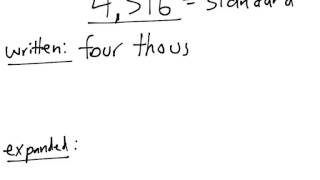By Freckle education

Read and write multi-digit whole numbers using base-ten numerals, number names, and expanded form# Understanding Numbers and Place Value (4.NBT.2)

##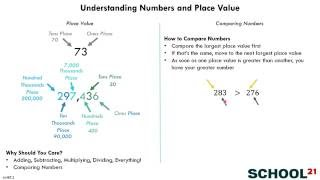By School 21

Read and write multi-digit whole numbers using base-ten numerals, number names, and expanded form# Expanded Form

##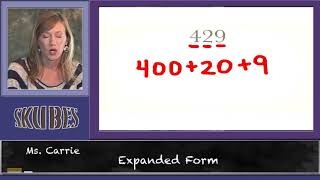By Skubes ed

Read and write multi-digit whole numbers using base-ten numerals, number names, and expanded form# Fractions on the Number Line

##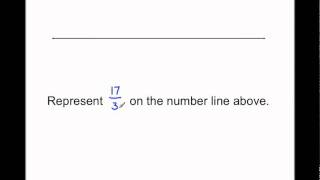By MathwithMrAlmeida

In this Singapore math lesson Mr. Almeida represents fractions on the number line by first defining how many parts make 1 whole. Then naming each part a fractional unit and counting that many units. This illustrates Common Core math standard 3.NF2.b.# [6.EE.4-1.0] Identify Equivalent Expressions - Common Core Standard

##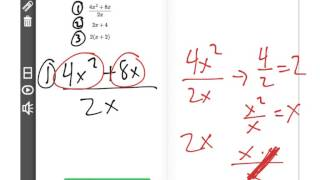By Freckle by Renaissance

Identify when two expressions are equivalent (i.e., when the two expressions name the same number regardless of which value is substituted into them). For example, the expressions y + y + y and 3y are equivalent because they name the same number regardless of which number y stands for. Front Row is a free, adaptive, Common Core aligned math program for teachers and students in kindergarten through eighth grade. Front Row allows students to practice math at their own pace - learning advanced concepts when they're ready and receiving remediation when they struggle. Front Row provides teachers with access to a detailed data dashboard and weekly email reports that show which standards are causing students difficulty, what small groups can be formed for interventions, and how their students are progressing in math.# Solving problems with percent

##By MathPlanetVideos

Solve "54 is 25% of what number?"# Proportions and percent

##By MathPlanetVideos

Write in percent or as decimals# Solving problems with percent

##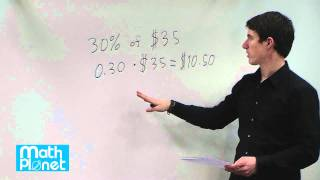By MathPlanetVideos

A skirt cost \$35 regulary in a shop. At a sale the price of the skirtreduces with 30%. How much will the skirt cost after the discount?# Math Antics - Negative Numbers

##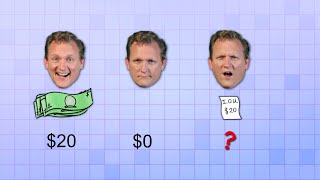By mathantics# Percent word problems 1 example 2)

##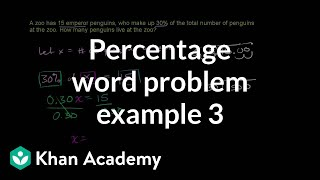By Khan Academy

You're given the percent in this example and asked to find the whole number represented by the percent.# Percent Change

##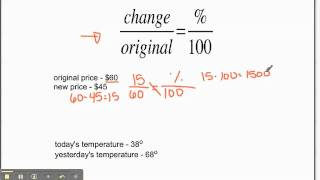By Mathademics

Calculating percentage change.# Describing the Meaning of Percent Part 2 of 2

##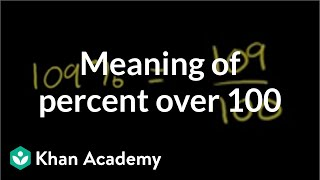By Khan Academy

This instructor in this video Sal Khan discusses how to solve this problem: Show 109 percent by shading the squares Mr. Khan uses computer software (with different colors) to illustrate his points. Sal Khan is the recipient of the 2009 Microsoft Tech Award in Education. The viewer may want to open the video to 'full screen' as the instructor fits a lot of information on a dark screen.# 15 - Complex Numbers & the Complex Plane

##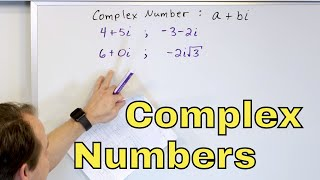By Math and Science

Quality Math And Science Videos that feature step-by-step example problems!# Percent to Decimal - YourTeacher.com - Math Help

##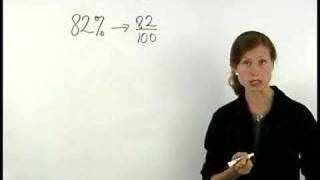By yourteachermathhelp

For a complete lesson on converting percents to decimals go to http://www.yourteacher.com - 1000+ online math lessons featuring a personal math teacher inside every lesson! In this lesson students learn to write percents as decimals and decimals as percents. For example to write 82% as a decimal think of 82% as 82/100 and remember that dividing by 100 moves the decimal point two places to the left to get 0.82. So 82% can be written as the decimal 0.82. Since a percent can be written as a decimal by moving the decimal point two places to the left a decimal can be written as a percent by moving the decimal point two places to the right. For example to write 0.4 as a percent move the decimal point two places to the right to get 40. So 0.4 can be written as 40%# Math Antics - Prime Factorization

##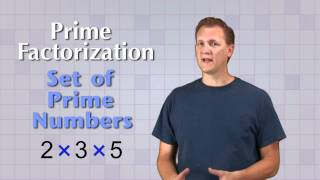By mathantics# Math Antics - Adding and Subtracting Fractions

##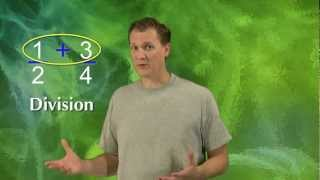By mathantics# Math Antics - Rounding

##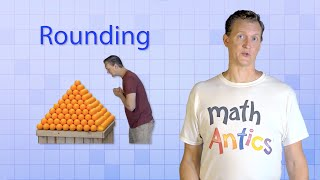By# The Basics of Organic Nomenclature: Crash Course Organic Chemistry #2

##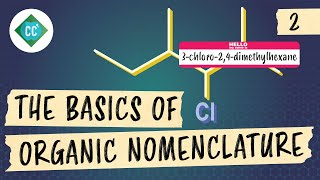By CrashCourse# Math Antics - Place Value

##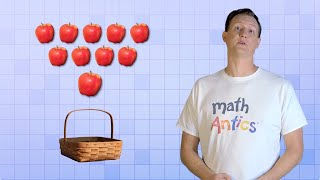By mathantics# Math Antics - Fractions and Decimals

##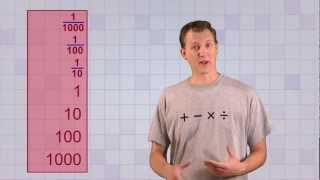By mathantics